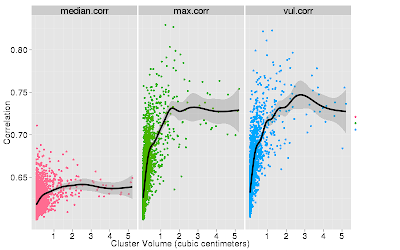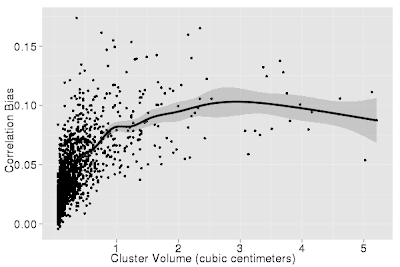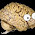## Tuesday, February 17, 2009

### Simulating Voodoo Correlations: How much voodoo, exactly, are we dealing with?

The recent article "Voodoo Correlations in Social Neuroscience" by Ed Vul and colleagues has gotten a lot of attention and has stimulated a great deal of discussion about statistical practices in functional neuroimaging. The main critique in the article by Vul involves a bias incurred when a correlation coefficient is re-computed by averaging over a cluster of active voxels that are selected from a whole-brain correlation analysis. Vul et al. correctly point out that the method will produce inflated estimates of the correlation magnitude. There have been several excellent replies to the original paper, including a detailed statistical rebuttal showing that the actual bias incurred by the two-stage correlation (henceforth: vul-correlation) is rather modest.

It occurred to me in thinking about this problem that the bias in the correlation magnitude should be related to the number voxels included in the selected cluster. For instance, in the case of a 1 voxel cluster the bias is obviously zero since there is only one voxel to average over. How fast does this bias increase as a function of cluster volume in a typical fMRI data set with a typically complex spatial covariance structure? Consideration of the high correlation among voxels within a cluster led me to wonder about the true extent of bias in vul-correlations. For instance, in the most extreme case, where all voxels in a cluster are perfectly correlated, there is zero inflation due to avergaing over voxels.

To explore these questions I ran some simulations with real world data. The data I used were from a study carried out on the old 4 Tesla magnet at UC Berkeley and consisted of a set of 27 spatially normalized and smoothed (7mm FWHM) contrasts in a verbal working memory experiment (delay period activation > baseline). The goal was to run many correlation analyses between the "real" contrast maps and a succession of randomly generated "behavioral scores". Thus, for each of 1000 iterations I sampled 27 values from a random normal distribution to create a set of random behavioral scores. I then computed the voxel-wise correlation between each set of scores with the set of 27 contrast maps. I then thresholded the resulting correlation maps at 0.6 (p = 0.001) and clustered the above-threshold voxels using FSL's "cluster" command. This resulted in 1000 thresholded (and clustered) statistical maps representing the correlation between a set of "real" contrast maps and 1000 randomly generated "behavioral scores".

Next, I loaded each of the 1000 statistical volumes and computed, for each active cluster, the minimum correlation in the cluster, the median correlation in the cluster, the maximum correlation in the cluster, and the two-stage vul-correlation. The vul-correlation was computed as follows: I extracted the matrix of values from the set of contrast maps for each cluster where (rows=number of subjects(27), columns=number of voxels in cluster) and averaged across columns, yielding a new vector of 27 values. I then recomputed the correlation coefficient between this averaged vector and the original randomly generated "behavioral variable" (all 1000 of which had been saved in a text file). Then I plotted cluster volume in cubic centimeters against its median, maximum, and vul-correlations. Here's the result.What you can see is that vul-correlation rapidly increases as a function of cluster volume, reaching asymptote at a correlation of about .73 and a cluster volume of roughly 2 cubic centimeters. You can see, however, that the maximum correlation, which is not a two-stage correlation, has almost the exact same functional profile. The median correlation within a cluster also increases somewhat, but not as high or as rapidly as the vul- and maximum- correlations.

To quantify the "bias" in the vul-correlation as a function of cluster size I plotted the difference between the vul-correlation and median correlation.It is clear from this plot that the bias becomes maximal when the cluster size is approximately 3 cubic centimeters. That is, however, rather a large cluster by fMRI standards. For a 1 cubic centimeter cluster the bias is about .075 and for a 1/2 cubic centimeter cluster (approximately 20 3 x 3 x 3 mm voxels) the bias is about 0.06. I'm not sure whether that rises to the level of "voodoo". Perhaps voodoo of a Gilligan's Island variety. Minor voodoo, if you like.

Lastly, I examined the minimum correlation as a function of cluster size. Of course, the minimum correlation can never fall below the cluster threshold, which was .6. Thus, I thought that the minimum correlation might serve as a good lower bound for reporting correlation magnitudes. You can see from the plot below that for these random simulations, at least, the minimum correlation does not increase with cluster size. In fact, it tends to approach the correlation threshold, which is not surprising, as this is what would be expected in a noise distribution. This time I've plotted cluster volume on a log (base 2) scale for easier visualization of the trend.So, what have I learned from this exercise? First, the amount of inflation incurred from a two-stage correlation (vul-corrrelation) increases as a function of cluster size. For smallish clusters (1/2 to 1 cubic centimeters) this bias is not that much, whereas for larger clusters the bias is as high as 0.1. Second, the maximum correlation has a nearly identical relation with cluster volume as does the vul-correlation. Finally, candidates for the reporting of cluster magnitudes could be the median or minimum correlations. The median correlation increases with cluster size, but not by much. The minimum correlation decreases with cluster size, but again not by much.

All in all, I think the problem identified by Vul et al. is a genuine one. Two-stage correlation estimates are inflated when compared to the median correlation within the cluster -- but not by that much. One reason for this is the high threshold required to achieve significance in whole-brain analyses yield voxels that don't have much room to go up. In addition, the constiuent voxels of a cluster are already highly correlated, so that the "truncation of the noise distribution" referred to by Vul et al. may be less than would be expected among truly independent voxels. So, perhaps, in the end the vul-correlation isn't so much a voodoo correlation as it is a vehicle for voodoo fame.

1.This is fascinating stuff. I also suspected that the magnitude of the inflation (the "voodoo factor" if you like) would be related to the number of voxels selected, but I didn't realize that it also depends upon the size of the cluster itself. And obviously I was too lazy to actually do any simulations. Nice work.

I know what Vul's response to your arguments would be, though.

He'd say that while the degree of inflation over and above the median correlation in the selected cluster may be minor, the more serious source of inflation is the selection of the cluster itself.

Assume that the truth is that activity in the whole anterior cingulate is correlated with behaviour with median r=0.2. You search for a cluster where median r=0.6. You find one, somewhere within the anterior cingulate, and report it. But that cluster represents a chance finding.

Of course, correcting for multiple comparisons is meant to guard against such chance findings. But it's designed to guard against chance findings under the null hypothesis of no true effect (noise normally distributed with a mean of zero).

If there is a true effect, albeit a small one, then the likelihood of a chance finding of a large effect is much higher than under the null.

In other words, if you find a cluster after correcting for multiple comparisons, you can be 95% confident that there is some true effect there but not that confident about the size of the effect.

Such, at least, is my understanding.

2.thanks for the comment, neuroskeptic!

Yes, you're absolutely right about Vul's hypothetical response to these simulations. They do not actually get to the heart of the matter. More sophisticated simulations are needed for that. As I mentioned in the second post, I thought the "non-independence error" referred to cases involving two hypothesis tests when in fact it does not require that at all.

On the other hand, if reporting correlation effect sizes in whole-brain analyses is at all justified (and many argue it is) then it will be helpful to know how these estimates vary as a function of the summary measure used and cluster size. At the very least, it seems that reporting the mean of median correlation is a better option than the peak.

3.It doesn't necessarily involve two hypothesis tests but it does involve two different "steps" - albeit only the first step is a formal statistical one.

In fact the whole Vul controversy is an excellent example of the fact that it's often the informal aspects of statistics that are the most problematic. You can get all your sums formally correct and still mislead, even unintentionally.